﻿ 一类离散种群模型的动力学性质 Dynamic Properties of a Class of Discrete Population Model

Vol. 08  No. 08 ( 2019 ), Article ID: 31806 , 8 pages
10.12677/AAM.2019.88171

Dynamic Properties of a Class of Discrete Population Model

Yuqing Chen, Yuman Zhang, Jiangming Xu, Xiaoliang Zhou

School of Mathematics and Statistics, Lingnan Normal University, Zhanjiang Guangdong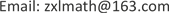Received: July 31st, 2019; accepted: August 15th, 2019; published: August 22nd, 2019ABSTRACT

In this paper, the dynamic behavior of a class of discrete population models is studied. Fixed point types and hyperbolic properties are determined by discussing coefficient parameters. By using central manifold theorem and bifurcation theory, we obtain the conditions of transcritical bifurcation and flip bifurcation at two fixed points.

Keywords:Discrete Population Model, Central Manifold Theorem, Transcritical Bifurcation, Flip Bifurcation1. 引言

$x\left(t\right)$$y\left(t\right)$ 分别表示在时间 $t=0,1,2,\cdots$ 时活跃种群和被动种群的密度。Newman等人在  中提出的活跃种群和被动种群之间的离散模型如下

$\left\{\begin{array}{l}x\left(t+1\right)=\left(1-\epsilon \right)f\left(x\left(t\right)\right)+\epsilon y\left(t\right),\\ y\left(t+1\right)=\left(1-\epsilon \right)y\left(t\right)+\epsilon f\left(x\left(t\right)\right),\\ x\left(0\right)\ge 0,y\left(0\right)\ge 0,x\left(0\right)+y\left(0\right)>0,\end{array}$

$\left\{\begin{array}{l}x\left(t+1\right)=\frac{\left(1-\epsilon \right)\gamma x\left(t\right)}{1+kx\left(t\right)}+\epsilon y\left(t\right),\\ y\left(t+1\right)=\left(1-\epsilon \right)y\left(t\right)+\frac{\epsilon \gamma x\left(t\right)}{1+kx\left(t\right)},\end{array}$ (1)

 (2)

2. 双曲和非双曲情形

2.1. 不动点 ${E}_{0}\left(0,0\right)$ 的性质

${\gamma }_{1}^{*}=\frac{1+{\epsilon }_{1}}{1-3{\epsilon }_{1}},{\epsilon }_{1}=1-\epsilon ,{l}_{1}=\left\{\left(\gamma ,{\epsilon }_{1}\right)|0<{\epsilon }_{1}<1,\gamma =1\right\},{l}_{2}=\left\{\left(\gamma ,{\epsilon }_{1}\right)|0<{\epsilon }_{1}<\frac{1}{3},\gamma ={\gamma }_{1}^{*}\right\},$

${B}_{2}=\left\{\left(\gamma ,{\epsilon }_{1}\right)|1<\gamma <{\gamma }_{1}^{*},0<{\epsilon }_{1}<\frac{1}{3}\right\},{B}_{3}=\left\{\left(\gamma ,{\epsilon }_{1}\right)|\gamma >{\gamma }_{1}^{*},0<{\epsilon }_{1}<\frac{1}{3}\right\}.$

1) 不动点 ${E}_{0}$ 是非双曲的，当且仅当 $\left(\gamma ,{\epsilon }_{1}\right)$ 位于直线 ${l}_{1,2}$ 上；

2) 如果 $\left(\gamma ,{\epsilon }_{1}\right)\in {B}_{0}$ ，该不动点是渐近稳定的；

3) 如果 $\left(\gamma ,{\epsilon }_{1}\right)\in {B}_{1,2}$ ，该不动点是一个鞍点；如果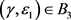，该不动点为不稳定的结点。

2.2. 不动点 ${E}_{1}\left({x}^{\ast },{x}^{\ast }\right)$ 的性质

${\gamma }_{2}^{*}=\frac{3\epsilon -2}{2-\epsilon },{\mathcal{D}}_{1}=\left\{\left(\gamma ,\epsilon \right)|0<\epsilon <1,\gamma =1\right\},{\mathcal{D}}_{2}=\left\{\left(\gamma ,\epsilon \right)|\frac{2}{3}<\epsilon <1,\gamma ={\gamma }_{2}^{*}\right\},$

${\mathcal{K}}_{1}=\left\{\left(\gamma ,\epsilon \right)|0<\epsilon \le \frac{2}{3},\gamma >1\right\},{\mathcal{K}}_{2}=\left\{\left(\gamma ,\epsilon \right)|\frac{2}{3}<\epsilon <1,\gamma >1\right\},$

${\mathcal{K}}_{3}=\left\{\left(\gamma ,\epsilon \right)|0<\epsilon <1,{\gamma }_{2}^{*}<\gamma <1\right\},{\mathcal{K}}_{4}=\left\{\left(\gamma ,\epsilon \right)|\frac{2}{3}<\epsilon <1,0<\gamma <{\gamma }_{2}^{*}\right\}.$

1) 不动点 ${E}_{1}$ 是非双曲的，当且仅当 $\left(\gamma ,\epsilon \right)\in {\mathcal{D}}_{1,2}$

2) 如果 $\left(\gamma ,\epsilon \right)\in {\mathcal{K}}_{1,2}$ ，不动点 ${E}_{1}$ 是渐近稳定的；

3) 如果 $\left(\gamma ,\epsilon \right)\in {\mathcal{K}}_{3}$ ，不动点 ${E}_{1}$ 是鞍点；如果 $\left(\gamma ,\epsilon \right)\in {\mathcal{K}}_{4}$ ，不动点 ${E}_{1}$ 为不稳定结点。

3. 跨临界分岔和Flip分岔

3.1. ${E}_{0}\left(0,0\right)$ 处的跨临界分岔和Flip分岔

${f}_{1}={\epsilon }^{2}-{\epsilon }_{1}{\lambda }_{2};{f}_{2}={\epsilon }^{2}-{\epsilon }_{1}{\lambda }_{1};{f}_{3}={\epsilon }_{1}^{2};{f}_{4}=\frac{{\epsilon }^{2}}{{\left(1+S\right)}^{2}}={\left(1-\epsilon \right)}^{4}.$

$D{F}_{\gamma }\left({E}_{0}\right)=\left[\begin{array}{cc}{\epsilon }_{1}\gamma & \left(1-{\epsilon }_{1}\right)\\ \left(1-{\epsilon }_{1}\right)\gamma & {\epsilon }_{1}\end{array}\right],$

${\lambda }_{1}=\frac{{\epsilon }_{1}\left(1+\gamma \right)-\sqrt{{\left({\epsilon }_{1}+\gamma {\epsilon }_{1}\right)}^{2}-8{\epsilon }_{1}\gamma +4\gamma }}{2},{\lambda }_{2}=\frac{{\epsilon }_{1}\left(1+\gamma \right)+\sqrt{{\left({\epsilon }_{1}+\gamma {\epsilon }_{1}\right)}^{2}-8{\epsilon }_{1}\gamma +4\gamma }}{2},$

${\left(\epsilon ,{\lambda }_{1}-{\epsilon }_{1}\gamma \right)}^{\tau },{\left(\epsilon ,{\lambda }_{2}-{\epsilon }_{1}\gamma \right)}^{\tau },$ (3)

$\left[\begin{array}{c}x\\ y\end{array}\right]=\left[\begin{array}{cc}-\epsilon & -\epsilon \\ {\lambda }_{1}-{\epsilon }_{1}\gamma & {\lambda }_{2}-{\epsilon }_{1}\gamma \end{array}\right]\left[\begin{array}{c}u\\ v\end{array}\right],$ (4)

$\left[\begin{array}{c}u\\ v\\ \gamma \end{array}\right]↦\left[\begin{array}{l}{\lambda }_{1}u\\ {\lambda }_{2}v\\ \gamma \end{array}\right]+\frac{1}{{\lambda }_{2}-{\lambda }_{1}}\left[\begin{array}{c}{f}_{1}\gamma \left(u+v\right)+{f}_{3}{\gamma }^{2}\left(u+v\right)-\frac{{f}_{1}\gamma \left(u+v\right)+{f}_{3}{\gamma }^{2}\left(u+v\right)}{1+k\epsilon \left(u+v\right)}\\ -{f}_{2}\gamma \left(u+v\right)-{f}_{3}{\gamma }^{2}\left(u+v\right)+\frac{{f}_{2}\gamma \left(u+v\right)+{f}_{3}{\gamma }^{2}\left(u+v\right)}{1+k\epsilon \left(u+v\right)}\\ 0\end{array}\right].$ (5)

$\left(\gamma ,{\epsilon }_{1}\right)\in {l}_{1}$ 时，有 ${\lambda }_{2}=1,{\lambda }_{1}={\epsilon }_{1}+{\epsilon }_{1}\gamma -1$ ，由文献  的定理2.1.4可知，令 ${\gamma }_{1}=\gamma -1$ ，在  附近，不动点 $\left(u,v\right)=\left(0,0\right)$ 的稳定性与分岔可通过研究在中心流形上一参数族映射来确定，中心流形有如下形式：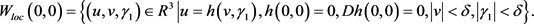$u=h\left(v,{\gamma }_{1}\right)=a{v}^{2}+bv{\gamma }_{1}+c{\gamma }_{1}^{2}+\mathcal{O}\left(3\right).$

$\begin{array}{l}\mathcal{N}\left(h\left(u,{\gamma }_{1}\right)\right)\\ =h\left(u,{\gamma }_{1}\right)-{\lambda }_{1}h\left(u,{\gamma }_{1}\right)-\frac{1}{{\lambda }_{2}-{\lambda }_{1}}\left\{{f}_{1}\left({\gamma }_{1}+1\right)\left[h\left(v,{\gamma }_{1}\right)+v\right]+{f}_{3}{\left({\gamma }_{1}+1\right)}^{2}\left[h\left(v,{\gamma }_{1}\right)+v\right]\begin{array}{c}\text{ }\\ \text{ }\end{array}\\ \text{\hspace{0.17em}}\text{\hspace{0.17em}}\text{ }-\frac{{f}_{1}\left({\gamma }_{1}+1\right)\left[h\left(u,{\gamma }_{1}\right)+v\right]+{f}_{3}{\left({\gamma }_{1}+1\right)}^{2}\left[h\left(u,{\gamma }_{1}\right)+v\right]}{1+k\epsilon \left[h\left(v,{\gamma }_{1}\right)+v\right]}\right\}=0.\end{array}$

$u=h\left(v,{\gamma }_{1}\right)=\mathcal{O}\left(3\right).$

$v↦{\Phi }_{{\gamma }_{1}}\left(v\right)={\lambda }_{2}v+\frac{1}{{\lambda }_{2}-{\lambda }_{1}}\left[-{f}_{2}\left({\gamma }_{1}+1\right)v-{f}_{3}{\left({\gamma }_{1}+1\right)}^{2}v+\frac{{f}_{2}\left({\gamma }_{1}+1\right)v+{f}_{3}{\left({\gamma }_{1}+1\right)}^{2}v}{1+kv}\right]+\mathcal{O}\left(3\right).$时，验算如下式子成立：

$\frac{\partial {\Phi }_{{\gamma }_{1}}}{\partial {\gamma }_{1}}\left(0,0\right)=0,\frac{\partial {\Phi }_{{\gamma }_{1}}}{\partial v\partial {\gamma }_{1}}\left(0,0\right)\ne 0,\frac{{\partial }^{2}\Phi }{\partial {v}^{2}}\left(0,0\right)\ne 0.$ (6)

$v↦{\Phi }_{{\gamma }_{1}}\left(v\right)={\lambda }_{2}v+\frac{1}{{\lambda }_{2}-{\lambda }_{1}}\left[-{f}_{2}\left({\gamma }_{1}+1\right)v-{f}_{3}{\left({\gamma }_{1}+1\right)}^{2}v+\frac{{f}_{2}\left({\gamma }_{1}+1\right)v+{f}_{3}{\left({\gamma }_{1}+1\right)}^{2}v}{1+kv}\right]$

$\begin{array}{l}v↦{\Phi }_{{\gamma }_{2}}\left(v\right)={\lambda }_{2}v+\frac{1}{{\lambda }_{2}-{\lambda }_{1}}\left[-{f}_{2}\left({\gamma }_{2}+{\gamma }_{1}^{*}\right)v-{f}_{3}{\left({\gamma }_{2}+{\gamma }_{1}^{*}\right)}^{2}v\begin{array}{c}\text{ }\\ \underset{}{\overset{}{\text{ }}}\end{array}\\ \text{\hspace{0.17em}}\text{\hspace{0.17em}}\text{\hspace{0.17em}}\text{\hspace{0.17em}}\text{\hspace{0.17em}}\text{\hspace{0.17em}}\text{\hspace{0.17em}}\text{\hspace{0.17em}}\text{\hspace{0.17em}}\text{\hspace{0.17em}}\text{\hspace{0.17em}}\text{\hspace{0.17em}}\text{\hspace{0.17em}}\text{\hspace{0.17em}}\text{\hspace{0.17em}}\text{\hspace{0.17em}}\text{\hspace{0.17em}}\text{\hspace{0.17em}}\text{\hspace{0.17em}}\text{\hspace{0.17em}}+\frac{{f}_{2}\left({\gamma }_{2}+{\gamma }_{1}^{*}\right)v+{f}_{3}{\left({\gamma }_{2}+{\gamma }_{1}^{*}\right)}^{2}v}{1+kv}\right]+\mathcal{O}\left(3\right).\end{array}$

$\left(\gamma ,{\epsilon }_{1}\right)\in {l}_{2}$ 时，有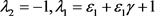，系统(1)在不动点 ${E}_{0}$

${\left[\frac{\partial {\Phi }_{{\gamma }_{2}}}{\partial {\gamma }_{2}}\frac{{\partial }^{2}{\Phi }_{{\gamma }_{2}}}{\partial {v}^{2}}+2\frac{{\partial }^{2}{\Phi }_{{\gamma }_{2}}}{\partial v\partial {\gamma }_{2}}\right]|}_{\left(0,0\right)}={\alpha }_{1}\ne 0,{\left[\frac{1}{2}{\left(\frac{{\partial }^{2}{\Phi }_{{\gamma }_{2}}}{\partial {v}^{2}}\right)}^{2}+\frac{1}{3}\frac{{\partial }^{3}{\Phi }_{{\gamma }_{2}}}{\partial {v}^{3}}\right]|}_{\left(0,0\right)}={\alpha }_{2}\ne 0.$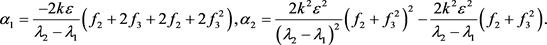3.2. ${E}_{1}\left({x}^{\ast },{x}^{\ast }\right)$ 的跨临界分岔和Flip分岔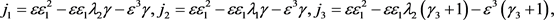${j}_{4}=\epsilon {\epsilon }_{1}^{2}-\epsilon {\epsilon }_{1}{\lambda }_{1}\left({\gamma }_{3}+1\right)-{\epsilon }^{3}\left({\gamma }_{3}+1\right),{j}_{5}=\epsilon {\epsilon }_{1}^{2}-\epsilon {\epsilon }_{1}{\lambda }_{2}\left({\gamma }_{4}+{\gamma }_{2}^{*}\right)-{\epsilon }^{3}\left({\gamma }_{4}+{\gamma }_{2}^{*}\right),$

${j}_{6}=\epsilon {\epsilon }_{1}^{2}-\epsilon {\epsilon }_{1}{\lambda }_{1}\left({\gamma }_{4}+{\gamma }_{2}^{*}\right)-{\epsilon }^{3}\left({\gamma }_{4}+{\gamma }_{2}^{*}\right),{j}_{7}={\epsilon }_{1}{\lambda }_{2}{\gamma }_{2}^{*}-{\epsilon }_{1}^{2}+{\epsilon }^{2}{\gamma }_{2}^{*}$

${j}_{8}=1+{e}^{*}\frac{3\epsilon -2}{2-\epsilon },{j}_{9}=\epsilon {\epsilon }_{1}{\lambda }_{2}+{\epsilon }^{3},e=\frac{2{j}_{4}}{\epsilon \left({\lambda }_{1}-{\lambda }_{2}\right)\left({\lambda }_{2}-1\right)},$

${e}^{*}=\frac{2\left(3\epsilon -2\right)\left(2-\epsilon \right){j}_{6}}{\epsilon {\lambda }_{2}\left({\lambda }_{1}-{\lambda }_{2}\right)\left({\lambda }_{2}-1\right){\left(3\epsilon -2\right)}^{2}-\epsilon {j}_{6}\left(8\epsilon -2\right)}.$

$D{\stackrel{˜}{F}}_{\gamma }\left({E}_{1}\right)=\left[\begin{array}{cc}\frac{{\epsilon }_{1}}{\gamma }& \epsilon \\ \frac{\epsilon }{\gamma }& {\epsilon }_{1}\end{array}\right],$

${\lambda }_{1}=\frac{\left({\epsilon }_{1}+{\epsilon }_{1}\gamma \right)\gamma -\sqrt{{\left({\epsilon }_{1}\gamma -{\epsilon }_{1}\right)}^{2}+4{\epsilon }^{2}\gamma }}{2\gamma },{\lambda }_{2}=\frac{\left({\epsilon }_{1}+{\epsilon }_{1}\gamma \right)\gamma +\sqrt{{\left({\epsilon }_{1}\gamma -{\epsilon }_{1}\right)}^{2}+4{\epsilon }^{2}\gamma }}{2\gamma },$

$\left[\begin{array}{c}u\\ v\\ \gamma \end{array}\right]↦\left[\begin{array}{c}{\lambda }_{1}u\\ {\lambda }_{2}v\\ \gamma \end{array}\right]+\frac{1}{\epsilon \left({\lambda }_{1}-{\lambda }_{2}\right)}\left[\begin{array}{c}\frac{{j}_{1}\left(u+v\right)}{1-k\epsilon \left(u+v\right)}-\frac{{j}_{1}\left(u+v\right)}{{\gamma }^{2}}\\ -\frac{{j}_{2}\left(u+v\right)}{1-k\epsilon \left(u+v\right)}+\frac{{j}_{2}\left(u+v\right)}{{\gamma }^{2}}\\ 0\end{array}\right].$ (7)

$T=\left[\begin{array}{cc}-\epsilon & -\epsilon \\ {\lambda }_{1}-\frac{{\epsilon }_{1}}{\gamma }& {\lambda }_{2}-\frac{{\epsilon }_{1}}{\gamma }\end{array}\right]$

${j}_{1}=\epsilon {\epsilon }_{1}^{2}-\epsilon {\epsilon }_{1}{\lambda }_{2}\gamma -{\epsilon }^{3}\gamma ,{j}_{2}=\epsilon {\epsilon }_{1}^{2}-\epsilon {\epsilon }_{1}{\lambda }_{1}\gamma -{\epsilon }^{3}\gamma .$

${\gamma }_{3}=\gamma -1$ ，假设在 ${\gamma }_{3}$ 附近的中心流形的形式为

$v=h\left(u,{\gamma }_{3}\right)=d{u}^{2}+eu{\gamma }_{3}+f{\gamma }_{3}^{2}+\mathcal{O}\left(3\right).$

$\mathcal{N}\left(h\left(u,{\gamma }_{3}\right)\right)=h\left(u,{\gamma }_{3}\right)-{\lambda }_{2}h\left(u,{\gamma }_{3}\right)+\frac{1}{\epsilon \left({\lambda }_{1}-{\lambda }_{2}\right)}\left\{\frac{{j}_{4}\left(u+h\left(u,{\gamma }_{3}\right)\right)}{1-k\epsilon \left(u+h\left(u,{\gamma }_{3}\right)\right)}-\frac{{j}_{4}\left(u+h\left(u,{\gamma }_{3}\right)\right)}{{\left({\gamma }_{3}+1\right)}^{2}}\right\}=0.$

$d=0,e=\frac{2{j}_{4}}{\epsilon \left({\lambda }_{1}-{\lambda }_{2}\right)\left({\lambda }_{2}-1\right)},f=0,$

$v=h\left(u,{\gamma }_{3}\right)=eu{\gamma }_{3}+\mathcal{O}\left(3\right).$ (8)

$\frac{\partial {\Phi }_{{\gamma }_{3}}}{\partial {\gamma }_{3}}\left(0,0\right)=0,\frac{\partial {\Phi }_{{\gamma }_{3}}}{\partial u\partial {\gamma }_{3}}\left(0,0\right)\ne 0,\frac{{\partial }^{2}{\Phi }_{{\gamma }_{3}}}{\partial {u}^{2}}\left(0,0\right)\ne 0.$

${e}^{*}=\frac{2\left(3\epsilon -2\right)\left(2-\epsilon \right){j}_{6}}{\epsilon {\lambda }_{2}\left({\lambda }_{1}-{\lambda }_{2}\right)\left({\lambda }_{2}-1\right){\left(3\epsilon -2\right)}^{2}-\epsilon {j}_{6}\left(8\epsilon -2\right)}.$

${\Phi }_{{\gamma }_{4}}\left(u\right)={\lambda }_{1}u+\frac{1}{\epsilon \left({\lambda }_{1}-{\lambda }_{2}\right)}\left[\frac{{j}_{5}\left(u+{e}^{*}u{\gamma }_{4}\right)}{1-k\epsilon \left(u+{e}^{*}u{\gamma }_{4}\right)}-\frac{{j}_{5}\left(u+{e}^{*}u{\gamma }_{4}\right)}{{\left({\gamma }_{4}+\frac{3\epsilon -2}{2-\epsilon }\right)}^{2}}\right]+\mathcal{O}\left(3\right).$

${\left[\frac{\partial {\Phi }_{{\gamma }_{4}}}{\partial {\gamma }_{4}}\frac{{\partial }^{2}{\Phi }_{{\gamma }_{4}}}{\partial {u}^{2}}+2\frac{{\partial }^{2}{\Phi }_{{\gamma }_{4}}}{\partial u\partial {\gamma }_{4}}\right]|}_{\left(0,0\right)}={\alpha }_{3}\ne 0,{\left[\frac{1}{2}{\left(\frac{{\partial }^{2}{\Phi }_{{\gamma }_{4}}}{\partial {u}^{2}}\right)}^{2}+\frac{1}{3}\frac{{\partial }^{3}{\Phi }_{{\gamma }_{4}}}{\partial {u}^{3}}\right]|}_{\left(0,0\right)}={\alpha }_{4}\ne 0.$

$\begin{array}{l}{\alpha }_{3}=-\frac{2}{\epsilon \left({\lambda }_{1}-{\lambda }_{2}\right)}\left\{2\epsilon {j}_{2}^{2}+k\epsilon {j}_{8}^{2}\left({j}_{9}+\epsilon {j}_{7}{j}_{8}\right)+{j}_{8}\left(2k{e}^{*}{\epsilon }^{2}{j}_{7}+{j}_{9}\right)+\frac{{j}_{9}-2{\epsilon }^{3}}{{\left(3\epsilon -2\right)}^{2}}\\ \text{\hspace{0.17em}}\text{\hspace{0.17em}}\text{\hspace{0.17em}}\text{\hspace{0.17em}}\text{\hspace{0.17em}}\text{\hspace{0.17em}}\text{ }-\frac{{\left(2-\epsilon \right)}^{2}\left(3{e}^{*}\epsilon -\epsilon -{e}^{*}+2\right)}{{\left(3\epsilon -2\right)}^{2}}+\frac{\left(2-\epsilon \right)\left[2\epsilon {\epsilon }_{1}^{2}+{e}^{*}\left(2-\epsilon \right)\right]+{j}_{9}}{{\left(3\epsilon -2\right)}^{3}}-\frac{\epsilon {\epsilon }_{1}^{2}\left(2-\epsilon \right)}{{\left(3\epsilon -2\right)}^{4}}\right\},\end{array}$

${\alpha }_{4}=\frac{2{k}^{2}{\epsilon }^{2}}{{\lambda }_{1}-{\lambda }_{2}}{j}_{8}^{2}\left[\frac{{j}_{7}^{2}}{{\lambda }_{1}-{\lambda }_{2}}-{j}_{5}{j}_{8}\right].$

4. 总结

Dynamic Properties of a Class of Discrete Population Model[J]. 应用数学进展, 2019, 08(08): 1463-1470. https://doi.org/10.12677/AAM.2019.88171

1. 1. Levin, S.A. (1974) Dispersion and Population Interactions. The American Naturalist, 108, 207-228. https://doi.org/10.1086/282900

2. 2. Newman, T.J., Antonovics, J., Wilbur, H.M., Newman, T.J. and Antonovics, J. (2002) Population Dynamics with a Refuge: Fractal Basins and the Suppression of Chaos. Theoretical Population Biology, 62, 121-128. https://doi.org/10.1006/tpbi.2002.1584

3. 3. Chow, Y. (2013) Coexistence in a Discrete Competition Model with Dispersal. Journal of Difference Equations & Applications, 19, 615-632. https://doi.org/10.1080/10236198.2012.663361

4. 4. Hassel, M.P. (1979) The Dynamics of Arthropod Preda-tor-Prey Systems. Princeton University Press, Vol. 35, 901-902

5. 5. Kapçak, S., Elaydi, S. and Ufuktepe, U. (2016) Stability of a Predator-Prey Model with Refuge Effect. Journal of Difference Equations & Applications, 22, 989-1004. https://doi.org/10.1080/10236198.2016.1170823

6. 6. Chow, Y. (2013) Coexistence in a Discrete Competition Model with Dispersal. Journal of Difference Equations & Applications, 19, 615-632.

7. 7. Rohani, P. and Ruxton, G.D. (1999) Dispersal and Stability in Metapopulations. Mathematical Medicine & Biology, 16, 297-306. https://doi.org/10.1093/imammb/16.3.297

8. 8. Silva, J. and Giordani, F. (2017) Density-Dependent Dispersal in Multiple Species Metapopulations. Mathematical Biosciences & Engineering, 5, 843-857. https://doi.org/10.3934/mbe.2008.5.843

9. 9. Abdul-Aziz, Y. (2008) Asynchronous and Synchronous Dispersals in Spatially Discrete Population Models. SIAM Journal on Applied Dynamical Systems, 7, 284-310. https://doi.org/10.1137/070688122

10. 10. Wiggins, S. (1990) Introduction to Applied Nonlinear Dynamical Systems and Chaos. 2th Edition, Springer-Verlag, New York. https://doi.org/10.1007/978-1-4757-4067-7

11. 11. Guckenheimer, J. and Holmes, P.J. (1983) Nonlinear Oscillations, Dynamical Systems and Bifurcations of Vector Fields. Springer-Verlag, New York. https://doi.org/10.1007/978-1-4612-1140-2